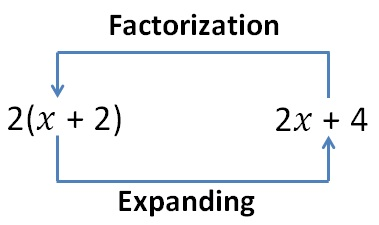Chapter 14 Class 8 Factorisation
Concept wiseHere,

We factorise 2x + 4 to get 2 (x + 2)

In this chapter, we will learn how to factorise by different methods

• Factorisation by common factor
• Factorisation by regrouping
• Factorisation by using identities
• Factorisation by splitting the middle term

## Factorization of Algebraic Expression

Algebraic expressions have numbers & variable in it

Example :

• 5xy
• 28 x 2 yz
• 41x
• 98y 2 z 4

Let’s learn how to factorise it

### Factorisation of 5xy

5xy = 5 × x × y

### Factorisation of 28x 2 yz

28x 2 yz = 2 × 2 × 7 × x 2 × y × z

= 2 × 2 × 7 × x × x × y × z

41x = 41 × x

### Factorisation of 98y 2 z 4

98y 2 z 4 = 2 × 7 × 7 × y 2 × z 4

= 2 × 7 × 7 × y × y × z × z × z × z

Get live Maths 1-on-1 Classs - Class 6 to 12